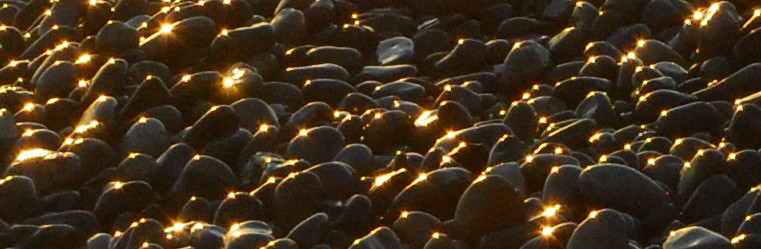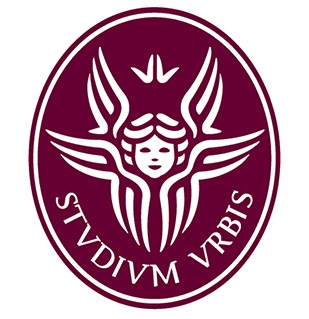Gabriella Puppo Dipartimento di Matematica
La Sapienza Universita' di Roma
Piazza Aldo Moro 5
00185 Roma
Italy• Numerical integration of PDEs
Many phenomena in physics are modelled with time dependent partial differential equations of hyperbolic type. These are usually nonlinear equations describing the propagation of signals with finite speeds, as in gas dynamics or shallow water flows, where the structures of interest are shocks or acoustic waves. But the same equations are also used for traffic flow, where the propagating signals are for instance the fronts of traffic jams. The research concentrates on the development of high order methods for the integration of such hyperbolic systems of equations. One topic concentrates on numerical methods able to guarantee the preservation of steady states for gas dynamic equations with a gravity field, or steady states in shallow water flows. Another topic of interest is in the development of numerical techniques for stiff regimes, especially low Mach flows, where the motion of a fluid is much slower than acoustic waves

• Computational methods and applications of kinetic models
Kinetic models arise when one wants to study the collective behaviour resulting from the interactions of particles. These techniques were initially formulated for rarefied gas dynamics, but they have been extended to describe also phenomena from social sciences or biology. The applications we study come from gas dynamics and traffic phenomena, with particular emphasis on the modelling of mixtures of particles of different kinds. The research concentrates both in the development of the model and in the creation of numerical techniques to compute the evolution of the solution, with particular emphasis on the preservation of correct asymptotic behaviours and the treatment of stiff regimes.